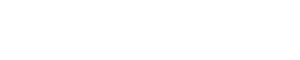# Fluid Machines

#### Course Topics

• Fluid Properties, Pressure and Its Measurement
• Different Properties,i.e Capillarity, Surface Tension, Viscosity
• Viscosity
• Newtonion and Non Newtonion Fluids
•  Non-Newtonian Fluids
• Surface Tension of Liquids
• Capillarity
• Vapour Pressure
• Pressure and its Measurement
• Pascal's Law
• Units and scales of Pressure Measurement
• Piezometer Tube
• The Barometer
• Manometers for measuring Gauge and Vacuum Pressure
• Manometers to measure Pressure Difference
• Inclined Tube Manometer
• Inverted Tube Manometer
• Different Types of Mechanical Gauges
• Hydrostatics
• Hydrostatic Thrusts on Submerged Curved Surfaces
• Buoyancy
• Stability of Unconstrained Submerged Bodies in Fluid
• Stable Equilibrium
• Unstable Equilibrium
• Neutral Equilibrium
• Stability of Floating Bodies in Fluid
• Period of Oscillation
• Fundamental of Fluid Flow and Equation of Motion
• Conservation of Energy
• pressure energy,potential energy,kinetic energy,total energy
• Euler's Equation of motion
• Bernoulli's Equation
• Measurement Of Flow Rate Through Pipe
• Venturimeter
• Orificemeter
• Flow Nozzle
• Stagnation Pressure
• Pitot Tube for Flow Measurement
• Flow Through Orifices And Mouthpieces
• Impacts of Jets and Jet Propulsion
• Force on a stationary surface
• Force on a moving surface
• Forces on Curve Surfaces due to the Impingement of Liquid Jets
• Propulsion of a Ship
• Jet Engine
• Flow and Losses in Pipes and Fittings
• Losses Due to Sudden Enlargement
• Losses Due to Sudden Contraction
• Entry and Exit Losses
• Losses In Pipe Bends
• Losses In Pipe Fittings
• Concept of Friction Factor in a pipe flow
• Variation of Friction Factor
• Pipes in Series
• Pipes in Parallel
• Power Transmission By A Pipeline

# Definition of Fluid

• A fluid is a substance that deforms continuously in the face of tangential or shear stress, irrespective of the   magnitude of shear stress .This continuous deformation under the application of shear stress constitutes a flow.
• In this connection fluid can also be defined as the state of matter that cannot sustain any shear stress.

Example : Consider Fig 1.1Fig 1.1 Shear stress on a fluid body

If a shear stress τ is applied at any location in a fluid, the element 011' which is initially at rest, will move to 022', then to 033'. Further, it moves to 044' and continues to move in a similar fashion.

In other words, the tangential stress in a fluid body depends on velocity of deformation and vanishes as this velocity approaches zero. A good example is Newton's parallel plate experiment where dependence of shear force on the velocity of deformation  was established.